# TRIM Function

Removes extra spaces in the data

## What is the TRIM Function?

The TRIM function is categorized under Excel Text functions. TRIM helps remove the extra spaces in data and thus clean up the cells in the worksheet.

In financial analysis, the TRIM function can be useful in removing irregular spacing from data imported from other applications.

### Formula

=TRIM(text)

1. Text (required argument) – This is the text from which we wish to remove spaces.

### A few notes about the TRIM Function:

• TRIM will remove extra spaces from text. Thus, it will leave only single spaces between words and no space characters at the start or end of the text.
• It is very useful when cleaning up text from other applications or environments.
• TRIM only removes the ASCII space character (32) from the text.
• The Unicode text often contains a non-breaking space character (160) that appears in web pages as an HTML entity. It will not be removed with TRIM.

### How to use the TRIM Function in Excel?

TRIM is a built-in function that can be used as a worksheet function in Excel. To understand the uses of the function, let’s consider a few examples:

#### Example 1

Suppose we are given the following data from an external source: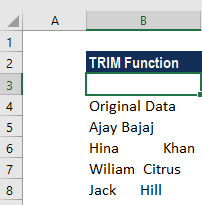For trimming the spaces, we will use the following function: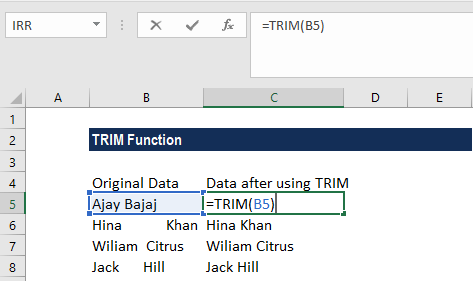We get the results below: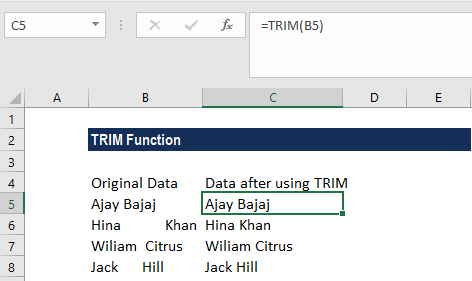#### Example 2

Let us see how the TRIM function works for numbers. Suppose we are the given the data below: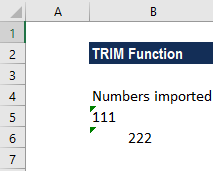The formula to use is: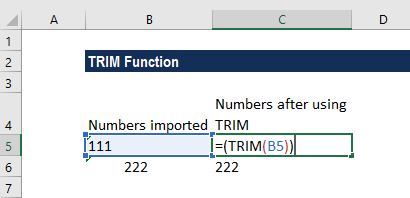Using the TRIM function, we will get the following result: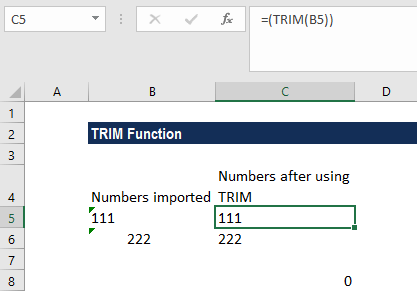As you can see above, the trimmed values are text strings, while we want numbers. To fix this, we will use the VALUE function along with the TRIM function, instead of the above formula: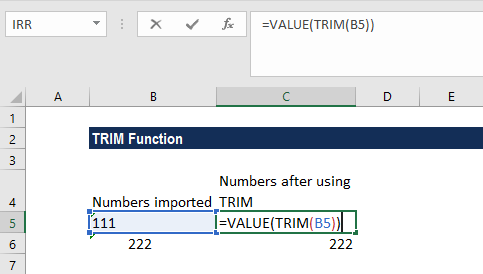The above formula removes all leading and trailing spaces, if any, and turns the resulting value into a number, as shown below: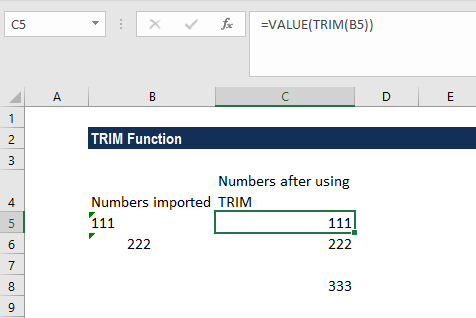#### Example 3

To join multiple cell values with a comma, we can use a formula based on the SUBSTITUTE and TRIM functions. Suppose we are given the following data: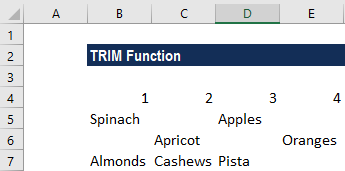The formula to use is: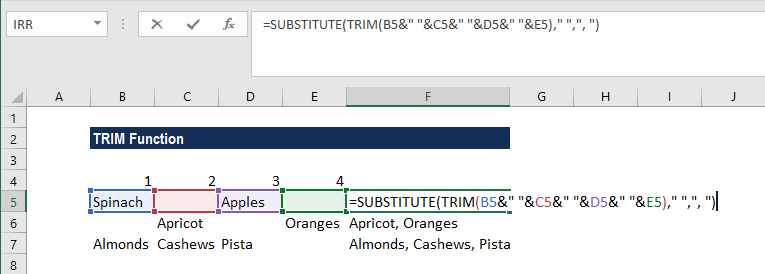The formula first joins the values in the four cells to the left using the concatenation operator (&) and a single space between each value. The TRIM function is used to “normalize” all spacing. TRIM automatically strips space at the start and end of a given string and leaves just one space between all words inside the string. It takes care of extra spaces caused by empty cells. SUBSTITUTE is used to replace each space (” “) with a comma and space (“, “). We used a delimiter to join cells.

We get the results below: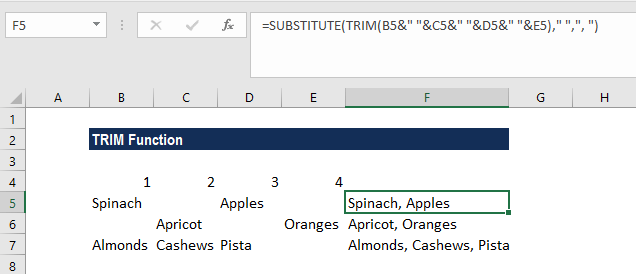Thanks for reading CFI’s guide to this important Excel function. By taking the time to learn and master these functions, you’ll significantly speed up your financial modeling. To learn more, check out these additional CFI resources:

• Excel Functions for Finance